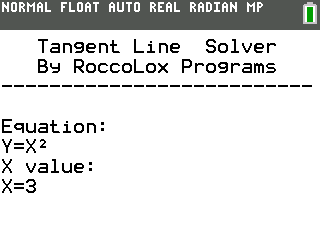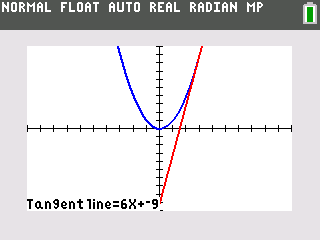Tangent Line Solver

## Description

A very simple yet useful program that just has you enter in an equation (in terms of Y=) and then
the X value of the tangent line. From there, the program will calculate the equation of the
tangent line for that equation and at the entered X value. This program will also store both equations
into the Y1 and Y2 variables of the calculator.

## Screenshots## Archive Contents

Name Size
TNGTLINE.8xp 692 bytes
File Size
985 bytes

2 years, 7 months ago

Rating
10/10 (1 vote)